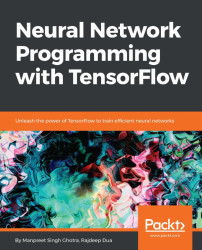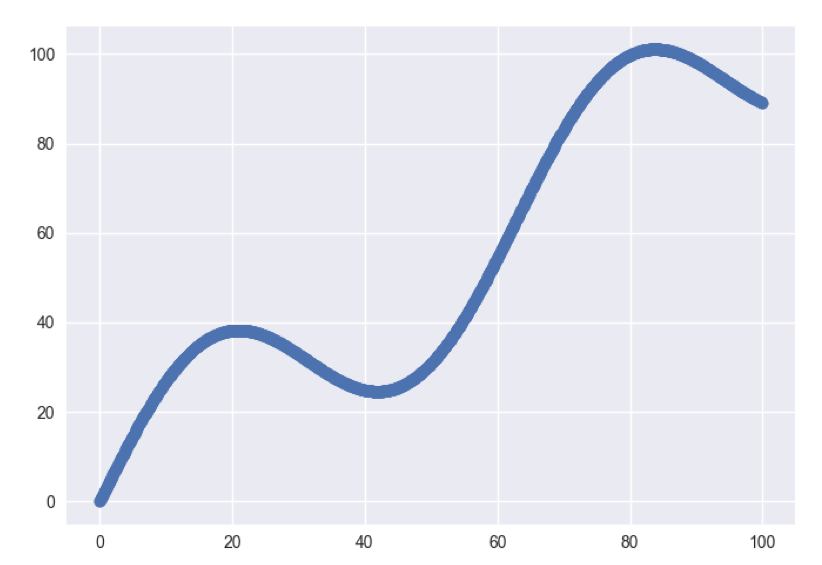•#### Neural Network Programming with Tensorflow#### Overview of this book

If you're aware of the buzz surrounding the terms such as "machine learning," "artificial intelligence," or "deep learning," you might know what neural networks are. Ever wondered how they help in solving complex computational problem efficiently, or how to train efficient neural networks? This book will teach you just that. You will start by getting a quick overview of the popular TensorFlow library and how it is used to train different neural networks. You will get a thorough understanding of the fundamentals and basic math for neural networks and why TensorFlow is a popular choice Then, you will proceed to implement a simple feed forward neural network. Next you will master optimization techniques and algorithms for neural networks using TensorFlow. Further, you will learn to implement some more complex types of neural networks such as convolutional neural networks, recurrent neural networks, and Deep Belief Networks. In the course of the book, you will be working on real-world datasets to get a hands-on understanding of neural network programming. You will also get to train generative models and will learn the applications of autoencoders. By the end of this book, you will have a fair understanding of how you can leverage the power of TensorFlow to train neural networks of varying complexities, without any hassle. While you are learning about various neural network implementations you will learn the underlying mathematics and linear algebra and how they map to the appropriate TensorFlow constructs.
Title PageCreditswww.PacktPub.comCustomer FeedbackPrefaceFree Chapter
Maths for Neural NetworksDeep Feedforward NetworksOptimization for Neural NetworksConvolutional Neural NetworksRecurrent Neural NetworksGenerative ModelsDeep Belief NetworkingAutoencodersResearch in Neural NetworksGetting started with TensorFlow## Which optimizer to choose

In the case that the input data is sparse or if we want fast convergence while training complex neural networks, we get the best results using adaptive learning rate methods. We also don't need to tune the learning rate. For most cases, Adam is usually a good choice.

### Optimization with an example

Let's take an example of linear regression, where we try to find the best fit for a straight line through a number of data points by minimizing the squares of the distance from the line to each data point. This is why we call it least squares regression. Essentially, we are formulating the problem as an optimization problem, where we are trying to minimize a loss function.

Let's set up input data and look at the scatter plot:

```# input data
xData = np.arange(100, step=.1)
yData = xData + 20 * np.sin(xData/10)```Define the data size and batch size:

```# define the data size and batch size
nSamples = 1000
batchSize = 100```

We will need to resize the data to meet the TensorFlow input format...